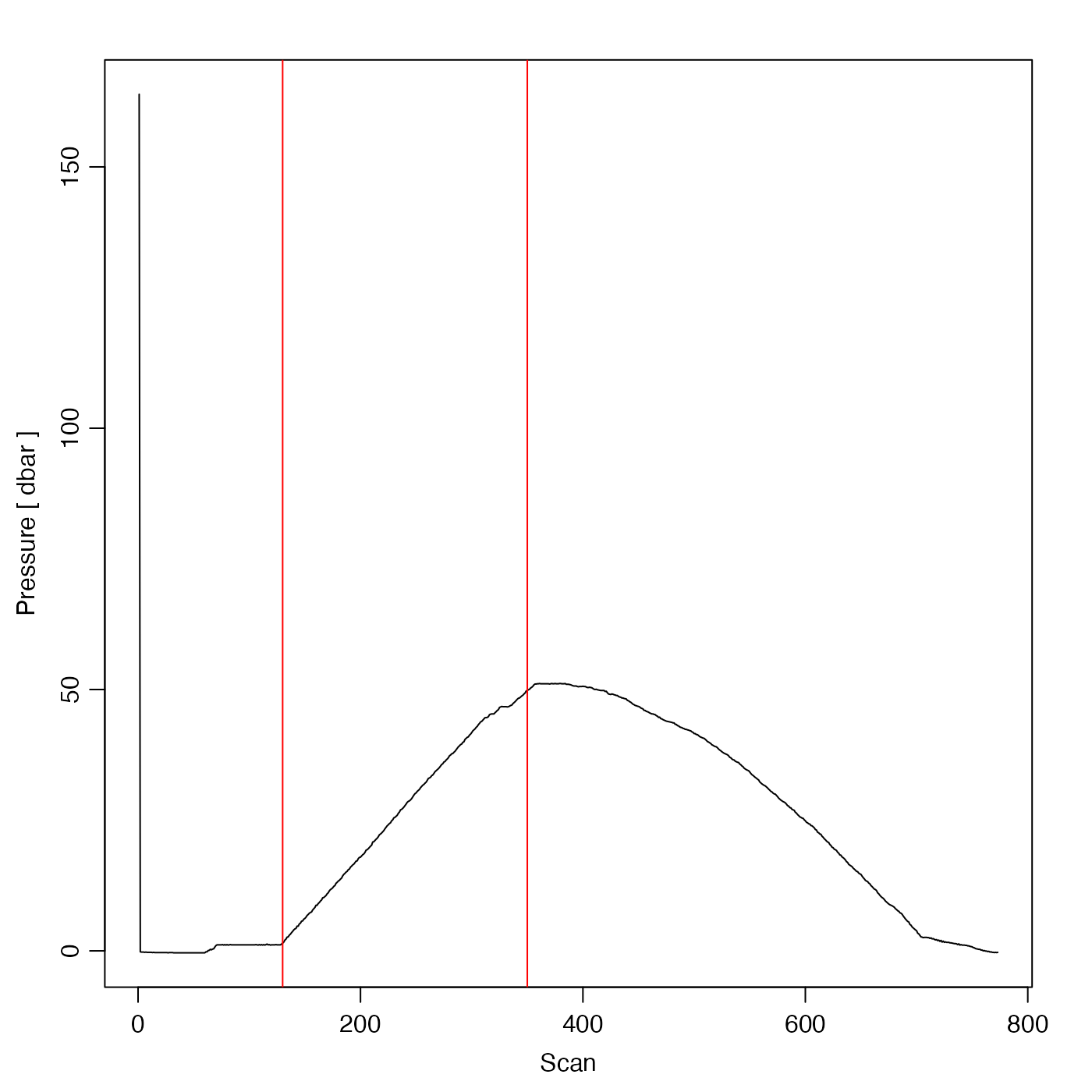Plot CTD data as time-series against scan number, to help with trimming extraneous data from a CTD cast.

plotScan(
x,
which = 1,
xtype = "scan",
flipy = FALSE,
type = "l",
mgp = getOption("oceMgp"),
xlim = NULL,
ylim = NULL,
mar = c(mgp + 1.5, mgp + 1.5, mgp, mgp),
...,
debug = getOption("oceDebug")
)

## Arguments

x

a ctd object.

which

integer specifying the plot to be drawn: 1 for pressure vs 'x', 2 for diff(pressure) vs 'x', 3 for temperature vs 'x', and 4 for salinity vs 'x' Here, the value of 'x' is determined by xtype.

xtype

Character string indicating variable for the x axis. The permitted values are "scan" (the default), "time" and "index". The last of these is created by using seq_along() on the pressure column (which is assumed to be present in any ctd object). Only xtype="index" is guaranteed to work for all objects, and indeed that value is used, if either "scan" or "time" is requested, but unavailable.

flipy

Logical value, ignored unless which is 1. If flipy is TRUE, then a pressure plot will have high pressures at the bottom of the axis.

type

Character indicating the line type, as for plot.default(). The default is "l", meaning to connect data with line segments. Another good choice is "o", to add points at the data.

mgp

Three-element numerical vector to use for par(mgp), and also for par(mar), computed from this. The default is tighter than the R default, in order to use more space for the data and less for the axes.

xlim

Limits on the x value. The default, NULL, is to select this from the data.

ylim

Limits on the y value. The default, NULL, is to select this from the data.

mar

Four-element vector be used with par("mar"). If set to NULL, then par("mar") is used. A good choice for a TS diagram with a palette to the right is mar=par("mar")+c(0, 0, 0, 1)).

...

Optional arguments passed to plotting functions.

debug

an integer specifying whether debugging information is to be printed during the processing. This is a general parameter that is used by many oce functions. Generally, setting debug=0 turns off the printing, while higher values suggest that more information be printed. If one function calls another, it usually reduces the value of debug first, so that a user can often obtain deeper debugging by specifying higher debug values.

## Historical Note

On 2022-12-07, xtype was expanded to include "index", and an undocumented multi-panel feature was removed.

Other functions that plot oce data: download.amsr(), plot,adp-method, plot,adv-method, plot,amsr-method, plot,argo-method, plot,bremen-method, plot,cm-method, plot,coastline-method, plot,ctd-method, plot,gps-method, plot,ladp-method, plot,landsat-method, plot,lisst-method, plot,lobo-method, plot,met-method, plot,odf-method, plot,rsk-method, plot,satellite-method, plot,sealevel-method, plot,section-method, plot,tidem-method, plot,topo-method, plot,windrose-method, plot,xbt-method, plotProfile(), plotTS(), tidem-class

Other things related to ctd data: CTD_BCD2014666_008_1_DN.ODF.gz, [[,ctd-method, [[<-,ctd-method, as.ctd(), cnvName2oceName(), ctd-class, ctd.cnv.gz, ctdDecimate(), ctdFindProfilesRBR(), ctdFindProfiles(), ctdRaw, ctdRepair(), ctdTrim(), ctd_aml.csv.gz, ctd, d200321-001.ctd.gz, d201211_0011.cnv.gz, handleFlags,ctd-method, initialize,ctd-method, initializeFlagScheme,ctd-method, oceNames2whpNames(), oceUnits2whpUnits(), plot,ctd-method, plotProfile(), plotTS(), read.ctd.aml(), read.ctd.itp(), read.ctd.odf(), read.ctd.odv(), read.ctd.saiv(), read.ctd.sbe(), read.ctd.ssda(), read.ctd.woce.other(), read.ctd.woce(), read.ctd(), setFlags,ctd-method, subset,ctd-method, summary,ctd-method, woceNames2oceNames(), woceUnit2oceUnit(), write.ctd()

Dan Kelley

## Examples

library(oce)
data(ctdRaw)
plotScan(ctdRaw)
abline(v=c(130, 350), col="red") # useful for ctdTrim()